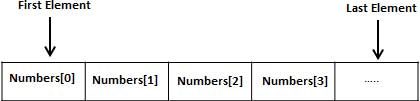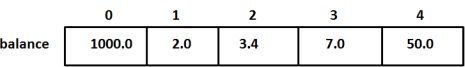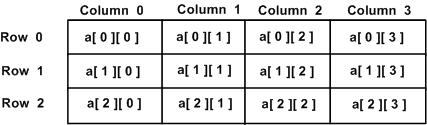# 数组• 有序性：数组元素之间具有固定的先后顺序
• 可索引：通过数组名和下标可以唯一地确定数组中的元素

# 声明数组

• 其中元素的数量为不可变量，C/C++ 不允许对数组的长度做动态定义。
• 数组名除了作为数组辨识名称之外，也表示了该数组存储空间的首地址，即数组名本身就是数组的内存入口地址，指向第一个元素。
• 一次只能使用数组中的单个元素，而不能一次使用整个数组。
// 正确用法：一次只能使用数组中的单个元素。
// 将 aArray 的数据复制到 bArray 中。
int aArray = {1, 2, 3, 4, 5};
int bArray = {0};
for(int i = 0; i < 5; i++)
{
bArray[i] = aArray[i];
}

// 错误用法：
int aArray = {1, 2, 3, 4, 5};
int bArray = {0};
bArray = aArray;    //不可给整个数组赋值


#include <stdio.h>
// 如下例所示，aArray[i*j] 在程序进行过程中，下标会超出其数组大小。
// 但是在编译和运行过程中，并不会报错，因此必须由编程人员对此边界进行处理！
int main(void)
{
int i, j;
int aArray = {1, 2, 3, 4, 5};
for (i = 0; i < 5; i++)
{
for (j = 0; j < 5; j++)
{
aArray[i * 5 + j] = i * 5 + j;
printf("aArray[%d]=%d\r\n", i * 5 + j, aArray[i * 5 + j]);
}
}
return 0;
}

/*
[root@c-dev ~]# ./main
aArray=0
aArray=1
aArray=2
aArray=3
aArray=4
aArray=5
aArray=32718
aArray=10
aArray=11
aArray=12
aArray=13
aArray=14
aArray=15
aArray=16
aArray=17
aArray=18
aArray=19
aArray=20
aArray=21
aArray=22
aArray=23
aArray=24

*/

// 上错误示例可改为：
// 当然像示例中的简单数组越界在编程过程中是十分容易避免的，但对于复杂度高的问题，必须是要增加边界检查的。
int main(void)
{
int i, j, idx;
int aArray = {1, 2, 3, 4, 5};
for (i = 0; i < 5; i++)
{
for (j = 0; j < 5; j++)
{
idx = i * 5 + j;
if (idx > sizeof(aArray) / sizeof(int))
{
printf("Err: idx over range!\r\nMax idx = %lu,idx=%d\r\n", sizeof(aArray) / sizeof(int), idx);
return 0;
}
aArray[idx] = idx;
printf("aArray[%d]=%d\r\n", idx , aArray[idx]);
}
}
return 0;
}

/*
[root@c-dev ~]# ./main
aArray=0
aArray=1
aArray=2
aArray=3
aArray=4
aArray=5
Err: idx over range!
Max idx = 5,idx=6
*/


# 初始化数据

• 指定数组长度的初始化：大括号 { } 之间的值的数目不能大于我们在数组声明时在方括号 [ ] 中指定的元素数目。
double balance = {1000.0, 2.0, 3.4, 7.0, 50.0};

• 不指定数组长度的初始化：数组的长度则为初始化时元素的个数。
double balance[] = {1000.0, 2.0, 3.4, 7.0, 50.0};

• 对指定的元素进行赋值
balance = 50.0;# 访问数组元素

double salary = balance;


# 二维数组

C 语言支持多维数组。多维数组声明的一般形式如下：

type name[size1][size2]...[sizeN];

int threedim;

• 二维数组int a = {
{0, 1, 2, 3} ,   /*  初始化索引号为 0 的行 */
{4, 5, 6, 7} ,   /*  初始化索引号为 1 的行 */
{8, 9, 10, 11}   /*  初始化索引号为 2 的行 */
};

//or

int a = {0,1,2,3,4,5,6,7,8,9,10,11};


int val = a;


# 指向数组的指针

double balance;


# 将数组指针作为实参传入函数

• 方式 1
void myFunction(int *param){}

• 方式 2
void myFunction(int param){}

• 方式 3
void myFunction(int param[]){}


#include <stdio.h>

/* 声明一个函数形参为整型指针类型 */
double getAvg(int arr[], int size);

int main(){
/* 定义并初始化一个数组变量 */
int balance = {1000, 2, 3, 17, 50};

/* 传递一个指向数组的指针作为函数实参 */
double avg = getAvg(balance, 5);
printf("AVG: %f", avg);
return 0;
}

double getAvg(int arr[], int size){
int i;
double avg;
double sum = 0;

for(i = 0; i < size; ++i){
sum += arr[i];
}

avg = sum / size;
return avg;
}


# 从函数返回一个数组指针

C 语言不允许函数返回一个完整的数组，但是可以返回一个指向数组的指针。注意，C 不支持在函数外部返回局部变量的地址，除非定义局部变量为 static 变量。

int * myFunction(){}


#include <stdio.h>
#include <stdlib.h>
#include <time.h>

/* 定义返回整型指针类型结果的函数 */
int * getRandom(){
static int r;
int i;

srand((unsigned)time(NULL));
for(i = 0; i < 10; ++i){
r[i] = rand();
printf("r[%d] = %d\n", i, r[i]);
}
return r;
}

int main(){
/* 定义一个整型指针变量 */
int *p;
int i;

p = getRandom();
for(i = 0; i < 10; i++){
printf("*(p + %d): %d\n", i, *(p + i));
}
return 0;
}


$./main r = 640773756 r = 1617898688 r = 2004130180 r = 494154148 r = 1999308605 r = 959614519 r = 81389324 r = 1893093458 r = 2121376870 r = 1095386666 *(p + 0): 640773756 *(p + 1): 1617898688 *(p + 2): 2004130180 *(p + 3): 494154148 *(p + 4): 1999308605 *(p + 5): 959614519 *(p + 6): 81389324 *(p + 7): 1893093458 *(p + 8): 2121376870 *(p + 9): 1095386666  # 指针数组 有一种情况，我们想用数组来存储指向 int 或 char 或其他数据类型的指针。下面是一个指向整数的指针数组的声明： int *ptr[MAX];  在这里，把 ptr 声明为一个数组，由 MAX 个整型指针组成。因此，ptr 中的每个元素，都是一个指向 int 值的指针。 #include <stdio.h> const int MAX = 3; int main () { int var[] = {10, 100, 200}; int i, *ptr[MAX]; for ( i = 0; i < MAX; i++) { ptr[i] = &var[i]; /* 将 int 值的地址赋值到整型指针数组 */ } for ( i = 0; i < MAX; i++) { printf("Value of var[%d] = %d\n", i, *ptr[i] ); } return 0; }  # 数组名和取数组首地址的区别 int array = {0};  以上述语句为例： • 数组名 array 本质是数组首元素的入口地址。 • 数组首元素的入口地址 &array • &array 取的是整个数组 array 的首地址。 虽然，&array&arrayarray 的数值相同，但本质却不同。这一点体现在 +1 偏移量上是非常明显的。 #include <stdio.h> int main() { int array = {0}; printf(" array = %p\n", array); printf(" &array = %p\n", &array); printf(" &array = %p\n", &array); printf("\n"); printf(" array + 1 = %p\n", array + 1); printf(" &array + 1 = %p\n", &array + 1); printf(" &array + 1 = %p\n", &array + 1); printf("\n"); printf(" sizeof(int) = %lu\n", sizeof(int)); printf(" sizeof(array) = %lu\n", sizeof(array)); printf(" sizeof(array) = %lu\n", sizeof(array)); printf(" sizeof(&array) = %lu\n", sizeof(&array)); printf("sizeof(&array) = %lu\n", sizeof(&array)); printf("\n"); return 0; }  运行： $ ./main
array = 0x7ffeecd8c830
&array = 0x7ffeecd8c830
&array = 0x7ffeecd8c830

array + 1 = 0x7ffeecd8c834
&array + 1 = 0x7ffeecd8c840
&array + 1 = 0x7ffeecd8c834

sizeof(int) = 4
sizeof(array) = 4
sizeof(array) = 16
sizeof(&array) = 8
sizeof(&array) = 8


1. 在 C 中，几乎所有使用数组的表达式中，数组名的值是个指针常量，也就是数组第一个元素的地址。 它的类型取决于数组元素的类型： 如果它们是 int 类型，那么数组名的类型就是 “指向 int 的常量指针“。
2. 在以下两种场合，数组名并不是用指针常量来表示：1）当数组名作为 sizeof 运算符操作数时，返回整个数组的长度，而不是指向数组的指针的长度；2）当数组名作为地址运算符 & 的操作数时，返回的是一个指向整个数组的指针，而不是指向数组首元素的指针。
3. 指针的 +1 是偏移量问题：一个类型为 X 的指针的移动，是以 sizeof(X) 为移动步进的。
1. array+1：在数组首元素的内存地址的基础上，偏移一个 sizeof(array) 单位。上例中，为 0x7fffe3743ae0 + 1 * sizeof(array) == 0x7fffe3743ae0 + 1 * sizeof(int) == 0x7fffe3743ae0 + 4 == 0x7fffe3743ae4
2. &array+1：在数组的首地址的基础上，偏移一个 sizeof(array) 单位。上例中，为 0x7fffe3743ae0 + 1 * sizeof(array) == 0x7fffe3743ae0 + 1 * sizeof(int) * 0x4 == 0x7fffe3743ae0 + 0x16 == 0x7fffe3743af0

05-312151

#### matlab中sum对矩阵求和以及size用法09-202万+

#### ElasticSearch java API - 聚合查询

04-06398

#### C 语言编程 — 高级数据类型 — void 类型

01-132127

#### 深入学习java源码之Double.parseDouble()与Double.valueOf()

08-22678

#### c语言数组的高级定义问题

03-08

07-06366

#### Go 语言编程 — 高级数据类型 — 数组

10-28231

#### MATLAB数据类型、数组

07-221819

#### 编程基础篇：编程语言基本常用数据类型的讨论。©️2020 CSDN 皮肤主题: 编程工作室 设计师: CSDN官方博客点击重新获取扫码支付1.余额是钱包充值的虚拟货币，按照1:1的比例进行支付金额的抵扣。
2.余额无法直接购买下载，可以购买VIP、C币套餐、付费专栏及课程。余额充值